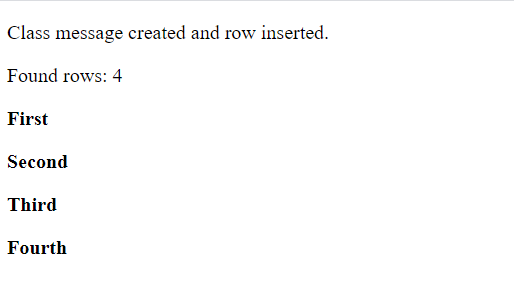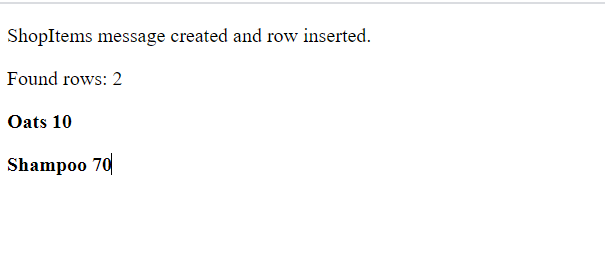# What is WEB SQL?

• Difficulty Level : Basic
• Last Updated : 30 May, 2022

Web SQL is a web page API for storing or managing the data in databases that can be queried using a variant of SQL like creating databases, opening the transaction, creating tables, inserting values to tables, deleting values, and reading data.

The Web SQL Database API is not a part of the HTML5 specification but it is a separate specification. It specifies a set of  APIs to manipulate the client-side databases using SQL. The web SQL database works in the latest version of Safari, Google Chrome, Android browsers, and Opera.

### Methods:

There are three basic methods as shown below as follows:

### Creating or Opening web SQL Database:

We can use the openDatabase function to create a database that has four parameters:

• Database name
• Version number
• Description
• Size
• Creation callback.

The creation callback gets called while the database is being created.

Use the openDatabase() method to access a database. If the database doesn’t exist, the method first creates it and then opens it:

`Syntax: var gfgDb = openDatabase(database name, version number, description, size);`

We can create a database by running the following query:

```var gfgDb = openDatabase('mydb', '1.0', 'this is a client side database', 2 * 1024 * 1024);

// To check whether the database is created or not.
if (!gfgDb) { alert('database not created'); }
else { var version = gfgDb.version; }```

### Creating transaction:

We can use the function called transaction from our database instance.

Syntax:

`gfgDb.transaction(function (tx){});`

Here, gfgDb is our database instance and tx is the transaction object that we will be using for upcoming operations. If any operation throws an error, the transaction will be rollbacked. Error logs can easily be managed by the use of transactions.

### Executing queries:

To execute a query you use the database.transaction() function.It has a single argument, that executes the query as below:

```var gfgDb = openDatabase('mydb', '1.0', 'Test DB', 2 * 1024 * 1024);

gfgDb.transaction(function (tx) {
tx.executeSql('CREATE TABLE IF NOT EXISTS CLASS (id unique, class)');
});  ```

The above code will create a table named CLASS in the ‘gfgDb’ database.

### INSERT Operation:

To create entries into the table as follows:

```var gfgDb = openDatabase('mydb', '1.0', 'Test DB', 2 * 1024 * 1024);

gfgDb.transaction(function (tx) {
tx.executeSql('CREATE TABLE IF NOT EXISTS CLASS (id unique, class)');
tx.executeSql('INSERT INTO CLASS (id, class) VALUES (1, "First")');
tx.executeSql('INSERT INTO CLASS (id, class) VALUES (2, "Second")');
}); ```

The second argument to executeSql maps field data to the query, like so:

```var id="1";
var text="First";

tx.executeSql('INSERT INTO CLASS (id, text) VALUES (?, ?)', [id, text]);```

Here, .id and text are external variables, and executeSql maps each item in the array argument to the “?”s.

To read already existing records we use a callback:

```var gfgDb = openDatabase('mydb', '1.0', 'Test DB', 2 * 1024 * 1024);

db.transaction(function (tx) {
tx.executeSql('CREATE TABLE IF NOT EXISTS CLASS (id unique, class)');
tx.executeSql('INSERT INTO CLASS (id, class) VALUES (1, "First")');
tx.executeSql('INSERT INTO CLASS (id, class) VALUES (2, "Second")');
});

db.transaction(function (tx) {
tx.executeSql('SELECT * FROM CLASS', [], function (tx, results) {
var len = results.rows.length, i;
msg = "<p>Found rows: " + len + "</p>";
document.querySelector('#status').innerHTML +=  msg;

for (i = 0; i < len; i++) {
}

}, null);
});```

The callback receives the transaction object and the results object. The results object contains a rows object, It has a length, but to reach individual rows, results.rows.item(i) is used, where i is the index of the row.

Example 1:

## HTML

 `  ``  ` `<``html``>   ``   ``<``head``>  ``    ` `      ``<``script` `type` `= ``"text/javascript"``>  ``         ``var gfgDb = openDatabase('mydb', '1.0', 'Test DB', 2 * 1024 * 1024);  ``         ``var msg;  ``      ` `         ``gfgDb.transaction(function (tx) {  ``            ``tx.executeSql('CREATE TABLE IF NOT EXISTS CLA (id unique, class,name)');  ``            ``tx.executeSql('INSERT INTO CLA (id, class,name) VALUES (1, "First","Geeks")');  ``            ``tx.executeSql('INSERT INTO CLA (id, class,name) VALUES (2, "Second","Hazel")'); ``            ``tx.executeSql('INSERT INTO CLA (id, class,name) VALUES (3, "Third","Ronaldo")'); ``            ``tx.executeSql('INSERT INTO CLA (id, class,name) VALUES (4, "Fourth","Sachin")'); ``             ` `            ``msg = '` `<``p``>Class message created and row inserted.` `';  ``            ``document.querySelector('#status').innerHTML =  msg;  ``         ``}) ``  ` `         ``gfgDb.transaction(function (tx) {  ``            ``tx.executeSql('SELECT * FROM CLA', [], function (tx, results) {  ``               ``var len = results.rows.length, i;  ``               ``msg = "` `<``p``>Found rows: " + len + "` `";  ``               ``document.querySelector('#status').innerHTML +=  msg;  ``        ` `               ``for (i = 0; i < ``len``; i++) {  ``                  ``msg = "` `

<``b``>" + results.rows.item(i).class + "` `";  ``                  ``document.querySelector('#status').innerHTML +=  msg;  ``               ``}  ``            ``}, null);  ``         ``});  ``      ``  ``   ``  ``    ` `   ``<``body``>  ``      ``<``div` `id` `= ``"status"` `name` `= ``"status"``>Status Message  ``   ``  `` `

Output:Example 2:

## HTML

 `  ``  ` `<``html``>   ``   ``<``head``>  ``    ` `      ``<``script` `type` `= ``"text/javascript"``>  ``         ``var gfgDb = openDatabase('mydb', '1.0', 'Test DB', 2 * 1024 * 1024);  ``         ``var msg;  ``      ` `         ``gfgDb.transaction(function (tx) {  ``            ``tx.executeSql('CREATE TABLE IF NOT EXISTS ShopItems (id unique, item_name, price)');  ``            ``tx.executeSql('INSERT INTO ShopItems (id, item_name, price) VALUES (1, "Oats","10")');  ``            ``tx.executeSql('INSERT INTO ShopItems (id, item_name, price) VALUES (2, "Shampoo","70")');  ``            ``msg = '` `<``p``>ShopItems message created and row inserted.` `';  ``            ``document.querySelector('#status').innerHTML =  msg;  ``         ``}) ``  ` `         ``gfgDb.transaction(function (tx) {  ``            ``tx.executeSql('SELECT * FROM ShopItems', [], function (tx, results) {  ``               ``var len = results.rows.length, i;  ``               ``msg = "` `<``p``>Found rows: " + len + "` `";  ``               ``document.querySelector('#status').innerHTML +=  msg;  ``        ` `               ``for (i = 0; i < ``len``; i++) {  ``                  ``msg = "` `

<``b``>" + results.rows.item(i).item_name +" "+ results.rows.item(i).price + "` `";  ``                  ``document.querySelector('#status').innerHTML +=  msg;  ``               ``}``           ` `            ``}, null);  ``         ``});  ``      ``  ``   ``  ``    ` `   ``<``body``>  ``      ``<``div` `id` `= ``"status"` `name` `= ``"status"``>Status Message  ``   ``  `` `

Output:My Personal Notes arrow_drop_up